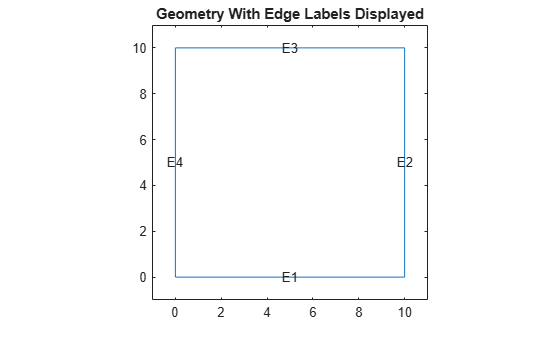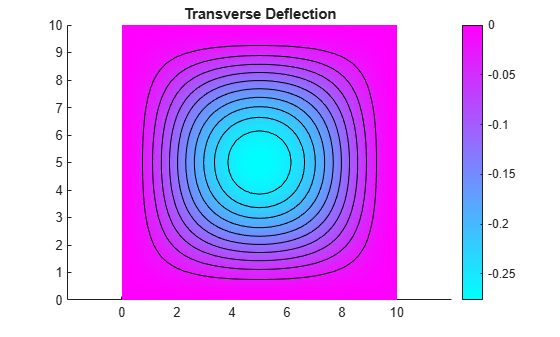Main Content

# Clamped Square Isotropic Plate with Uniform Pressure Load

This example shows how to calculate the deflection of a structural plate under a pressure loading.

The partial differential equation for a thin isotropic plate with a pressure loading is

`${\nabla }^{2}\left(D{\nabla }^{2}w\right)=-p,$`

where $D$ is the bending stiffness of the plate given by

`$D=\frac{E{h}^{3}}{12\left(1-{\nu }^{2}\right)},$`

and $E$ is the modulus of elasticity, $\nu$ is Poisson's ratio, $h$ is the plate thickness, $w$ is the transverse deflection of the plate, and $p$ is the pressure load.

The boundary conditions for the clamped boundaries are $w=0$ and ${w}^{\prime }=0$, where ${w}^{\prime }$ is the derivative of $w$ in a direction normal to the boundary.

Partial Differential Equation Toolbox™ cannot directly solve this fourth-order plate equation. Convert the fourth-order equation to these two second-order partial differential equations, where $v$ is the new dependent variable.

`${\nabla }^{2}w=v$`

`$D{\nabla }^{2}v=-p$`

You cannot directly specify boundary conditions for both $w$ and ${w}^{\prime }$ in this second-order system. Instead, specify that ${w}^{\prime }$ is 0, and define ${v}^{\prime }$ so that $w$ also equals 0 on the boundary. To specify these conditions, use stiff "springs" distributed along the boundary. The springs apply a transverse shear force to the plate edge. Define the shear force along the boundary due to these springs as $n\cdot D\nabla v=-kw$, where $n$ is the normal to the boundary, and $k$ is the stiffness of the springs. This expression is a generalized Neumann boundary condition supported by the toolbox. The value of $k$ must be large enough so that $w$ is approximately 0 at all points on the boundary. It also must be small enough to avoid numerical errors due to an ill-conditioned stiffness matrix.

The toolbox uses the dependent variables ${\mathit{u}}_{1}$ and ${\mathit{u}}_{2}$ instead of $w$ and $v$. Rewrite the two second-order partial differential equations using variables ${\mathit{u}}_{1}$ and ${\mathit{u}}_{2}$:

`$-{\nabla }^{2}{u}_{1}+{u}_{2}=0$`

`$-D{\nabla }^{2}{u}_{2}=p$`

Create a PDE model for a system of two equations.

`model = createpde(2);`

Create a square geometry and include it in the model.

```len = 10; gdm = [3 4 0 len len 0 0 0 len len]'; g = decsg(gdm,'S1',('S1')'); geometryFromEdges(model,g);```

Plot the geometry with the edge labels.

```figure pdegplot(model,'EdgeLabels','on') ylim([-1,11]) axis equal title 'Geometry With Edge Labels Displayed'```PDE coefficients must be specified using the format required by the toolbox. For details, see

The c coefficient in this example is a tensor, which can be represented as a 2-by-2 matrix of 2-by-2 blocks:

`$\left[\begin{array}{cccc}c\left(1\right)& c\left(2\right)& \cdot & \cdot \\ \cdot & c\left(3\right)& \cdot & \cdot \\ \cdot & \cdot & c\left(4\right)& c\left(5\right)\\ \cdot & \cdot & \cdot & c\left(6\right)\end{array}\right]$`

This matrix is further flattened into a column vector of six elements. The entries in the full 2-by-2 matrix (defining the coefficient a) and the 2-by-1 vector (defining the coefficient f) follow directly from the definition of the two-equation system.

```E = 1.0e6; % Modulus of elasticity nu = 0.3; % Poisson's ratio thick = 0.1; % Plate thickness pres = 2; % External pressure D = E*thick^3/(12*(1 - nu^2)); c = [1 0 1 D 0 D]'; a = [0 0 1 0]'; f = [0 pres]'; specifyCoefficients(model,'m',0,'d',0,'c',c,'a',a,'f',f);```

To define boundary conditions, first specify spring stiffness.

`k = 1e7;`

Define distributed springs on all four edges.

```bOuter = applyBoundaryCondition(model,'neumann','Edge',(1:4),... 'g',[0 0],'q',[0 0; k 0]);```

Generate a mesh.

`generateMesh(model);`

Solve the model.

`res = solvepde(model);`

Access the solution at the nodal locations.

`u = res.NodalSolution;`

Plot the transverse deflection.

```numNodes = size(model.Mesh.Nodes,2); figure pdeplot(model,'XYData',u(:,1),'Contour','on') title 'Transverse Deflection'```Find the transverse deflection at the plate center.

```numNodes = size(model.Mesh.Nodes,2); wMax = min(u(1:numNodes,1))```
```wMax = -0.2763 ```

Compare the result with the deflection at the plate center computed analytically.

`wMax = -.0138*pres*len^4/(E*thick^3)`
```wMax = -0.2760 ```

## SupportGet trial now